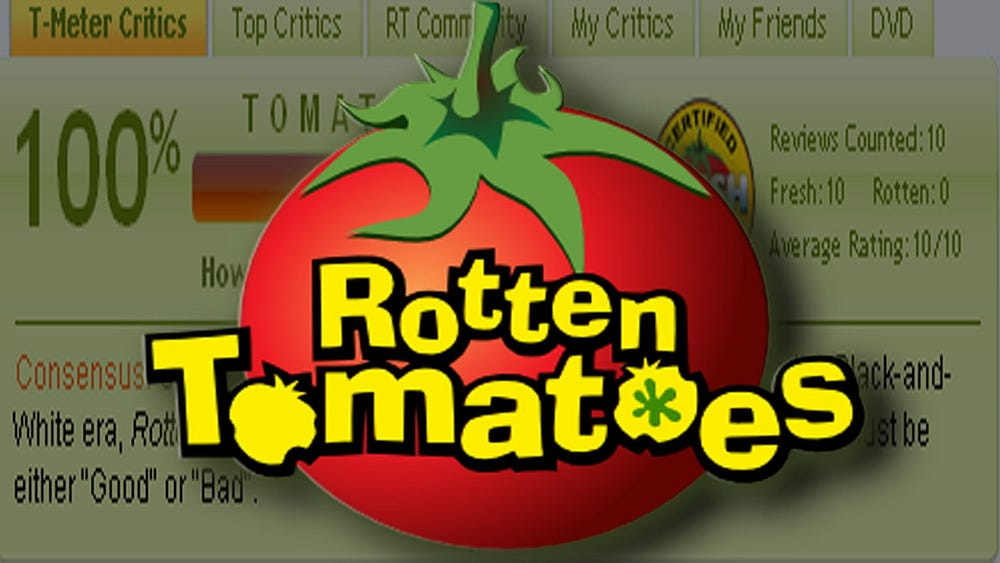# Multi label Text Classification — Rotten Tomatoes

## How many times we go to a movie after looking at the review…for me review makes a huge difference

Recently while I was exploring Kaggle, I found this interesting project on “Movie Review Sentiment Analysis ”. The task as mentioned by Kaggle: “Classify the sentiment of sentences from the Rotten Tomatoes data set”.

This is a multi-class classification problem, which simply means the data set have more than 2 classes(binary classifier). The five classes corresponding to sentiments:

The data set is quite challenging as the dataset is split into separate phrases and each one of them have a sentiment label. Moreover, obstacles like sentence negation, sarcasm, terseness, language ambiguity, and many others make this task very interesting for Natural Language Processing.

### Data Exploration

Let us have a look at first few phrases of the training dataset:

### Number of comments across categories

As we can see that the dataset is imbalanced, the dataset have to resampled so, that our model is not biased.

### Data Preprocessing

At first, the data needs to be cleaned which means we remove the punctuation marks, convert the data to lower case and also lemmatize the data. Lemmatizing means to group together variant forms of the same word.

After cleaning the data, the ‘Phrase’ column has been converted into the ‘clean-review’ column.

### n-grams and Tfidf Vectorizer

Since, we would like to differentiate between the words ‘good’ and ‘not good’ n-grams(1,2) is used, which would help to understand the context better as it would take into consideration both the words together, rather than a single word. Also, I have used Tfidf Vectorizer, which helps us to stop giving undue advantage to the stop words (since they are higher in frequency in a document) and gives more weightage to the contextual words.

### Machine Learning

#### Logistic Regression

For fitting the model with Logistic Regression, I have used OnevsRest classifier. This strategy consists in fitting one classifier per class. For each classifier, the class is fitted against all the other classes. This strategy is used for multilabel learning, where a classifier is used to predict multiple labels for instance, by fitting on a 2-d matrix in which cell [i, j] is 1 if sample i has label j and 0 otherwise.

The accuracy for this model is 72.17%. I have also used Linear SVC and Multinomial Naive Bayes algorithms for which mean accuracy were 77.44% and 59.50% respectively.

Since, deep learning works well with text data, I have decided to further analyze the data sets using various deep learning techniques.

### Deep Learning

#### Splitting Training data

The training data is split into training and validation data using sklearn train-test split library.

#### Data Preprocessing

After splitting the data, total number of features have been calculated, which is the total number of unique words in the corpus.

Once we got the maximum number of features, our next task is to find the maximum review length which is the total number of words in each review.

### Tokenizer

A neural network cannot work directly on text-strings so we must convert it somehow. There are two steps in this conversion, the first step is called the “tokenizer” which converts words to integers and is done on the data-set before it is input to the neural network. The second step is an integrated part of the neural network itself and is called the “embedding”-layer, which is described further below.

Deep learning libraries assume a vectorized representation of your data. In the case of variable length sequence prediction problems, this requires that the data be transformed such that each sequence has the same length.

After tokenizing and sequence padding we are ready to build our deep learning model.

### Create the Recurrent Neural Network

The first layer in the RNN is a so-called Embedding-layer which converts each integer-token into a vector of values. This is necessary because the integer-tokens may take on values between 0 and 10000 for a vocabulary of 10000 words. The RNN cannot work on values in such a wide range. The embedding-layer is trained as a part of the RNN and will learn to map words with similar semantic meanings to similar embedding-vectors.

The maximum number of features which we calculated before has been taken as input for the embedding layer.

I have used 3 different variants of RNN and compared the accuracy.

LSTM(Long-Short-Term-Memory)

This model gave an accuracy of around 71.94 % . Other two models I have used were CNN (Convoluted Neural Network) and CNN+GRU. The accuracy were 80.17% and 78.21%.

To test the models further, new data has been taken into consideration.

### Model Testing

This data is further processed (tokenize and sequence padding) for predicting with different RNN models.

1. LSTM